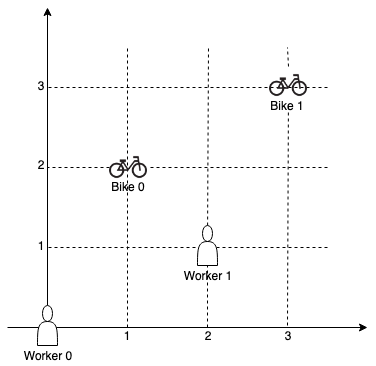##### Welcome to Subscribe On Youtube

Formatted question description: https://leetcode.ca/all/1057.html

# 1057. Campus Bikes

Medium

## Description

On a campus represented as a 2D grid, there are N workers and M bikes, with N <= M. Each worker and bike is a 2D coordinate on this grid.

Our goal is to assign a bike to each worker. Among the available bikes and workers, we choose the (worker, bike) pair with the shortest Manhattan distance between each other, and assign the bike to that worker. (If there are multiple (worker, bike) pairs with the same shortest Manhattan distance, we choose the pair with the smallest worker index; if there are multiple ways to do that, we choose the pair with the smallest bike index). We repeat this process until there are no available workers.

The Manhattan distance between two points p1 and p2 is Manhattan(p1, p2) = |p1.x - p2.x| + |p1.y - p2.y|.

Return a vector ans of length N, where ans[i] is the index (0-indexed) of the bike that the i-th worker is assigned to.

Example 1:Input: workers = [[0,0],[2,1]], bikes = [[1,2],[3,3]]

Output: [1,0]

Explanation:

Worker 1 grabs Bike 0 as they are closest (without ties), and Worker 0 is assigned Bike 1. So the output is [1, 0].

Example 2:Input: workers = [[0,0],[1,1],[2,0]], bikes = [[1,0],[2,2],[2,1]]

Output: [0,2,1]

Explanation:

Worker 0 grabs Bike 0 at first. Worker 1 and Worker 2 share the same distance to Bike 2, thus Worker 1 is assigned to Bike 2, and Worker 2 will take Bike 1. So the output is [0,2,1].

Note:

1. 0 <= workers[i][j], bikes[i][j] < 1000
2. All worker and bike locations are distinct.
3. 1 <= workers.length <= bikes.length <= 1000

## Solution

For each iteration, loop over the workers and loop over the bikes to find the shortest Manhattan distance between a worker and a bike. If there is a tie, always keep the smallest worker index and the smallest bike index. After the (worker, bike) pair is determined, assign the bike to the worker, and set both the worker and the bike as assigned. In the following iterations, if a worker or a bike is already assigned, then skip the worker or the bike. Continue the process until each worker is assigned a bike.

• class Solution {
public int[] assignBikes(int[][] workers, int[][] bikes) {
int workersLength = workers.length, bikesLength = bikes.length;
int[][] distances = new int[workersLength][bikesLength];
for (int i = 0; i < workersLength; i++) {
int[] worker = workers[i];
for (int j = 0; j < bikesLength; j++) {
int[] bike = bikes[j];
distances[i][j] = manhattanDistance(worker, bike);
}
}
int[] ret = new int[workersLength];
boolean[] workersAssigned = new boolean[workersLength];
boolean[] bikesAssigned = new boolean[bikesLength];
for (int i = 0; i < workersLength; i++) {
int minDistance = Integer.MAX_VALUE;
int minWorkerIndex = -1, minBikeIndex = -1;
for (int j = 0; j < workersLength; j++) {
if (workersAssigned[j])
continue;
for (int k = 0; k < bikesLength; k++) {
if (bikesAssigned[k])
continue;
int distance = distances[j][k];
if (distance < minDistance) {
minDistance = distance;
minWorkerIndex = j;
minBikeIndex = k;
}
}
}
ret[minWorkerIndex] = minBikeIndex;
workersAssigned[minWorkerIndex] = true;
bikesAssigned[minBikeIndex] = true;
}
return ret;
}

public int manhattanDistance(int[] point1, int[] point2) {
return Math.abs(point1 - point2) + Math.abs(point1 - point2);
}
}

• // OJ: https://leetcode.com/problems/campus-bikes/
// Time: O(MNlog(MN))
// Space: O(MN)
class Solution {
public:
vector<int> assignBikes(vector<vector<int>>& W, vector<vector<int>>& B) {
int N = W.size(), M = B.size();
vector<array<int, 3>> v;
auto dist = [](auto &a, auto &b) {
return abs(a - b) + abs(a - b);
};
for (int i = 0; i < N; ++i) {
for (int j = 0; j < M; ++j) {
v.push_back({dist(W[i], B[j]), i, j});
}
}
vector<bool> used(M);
sort(begin(v), end(v));
vector<int> ans(N, -1);
for (int i = 0, cnt = 0; i < v.size() && cnt < N; ++i) {
if (used[v[i]] || ans[v[i]] != -1) continue;
used[v[i]] = true;
ans[v[i]] = v[i];
++cnt;
}
return ans;
}
};

• class Solution:
def assignBikes(
self, workers: List[List[int]], bikes: List[List[int]]
) -> List[int]:
n, m = len(workers), len(bikes)
arr = []
for i, j in product(range(n), range(m)):
dist = abs(workers[i] - bikes[j]) + abs(workers[i] - bikes[j])
arr.append((dist, i, j))
arr.sort()
vis1 = [False] * n
vis2 = [False] * m
ans =  * n
for _, i, j in arr:
if not vis1[i] and not vis2[j]:
vis1[i] = vis2[j] = True
ans[i] = j
return ans


• func assignBikes(workers [][]int, bikes [][]int) []int {
n, m := len(workers), len(bikes)
type tuple struct{ d, i, j int }
arr := make([]tuple, n*m)
for i, k := 0, 0; i < n; i++ {
for j := 0; j < m; j++ {
d := abs(workers[i]-bikes[j]) + abs(workers[i]-bikes[j])
arr[k] = tuple{d, i, j}
k++
}
}
sort.Slice(arr, func(i, j int) bool {
if arr[i].d != arr[j].d {
return arr[i].d < arr[j].d
}
if arr[i].i != arr[j].i {
return arr[i].i < arr[j].i
}
return arr[i].j < arr[j].j
})
vis1, vis2 := make([]bool, n), make([]bool, m)
ans := make([]int, n)
for _, e := range arr {
i, j := e.i, e.j
if !vis1[i] && !vis2[j] {
vis1[i], vis2[j] = true, true
ans[i] = j
}
}
return ans
}

func abs(x int) int {
if x < 0 {
return -x
}
return x
}